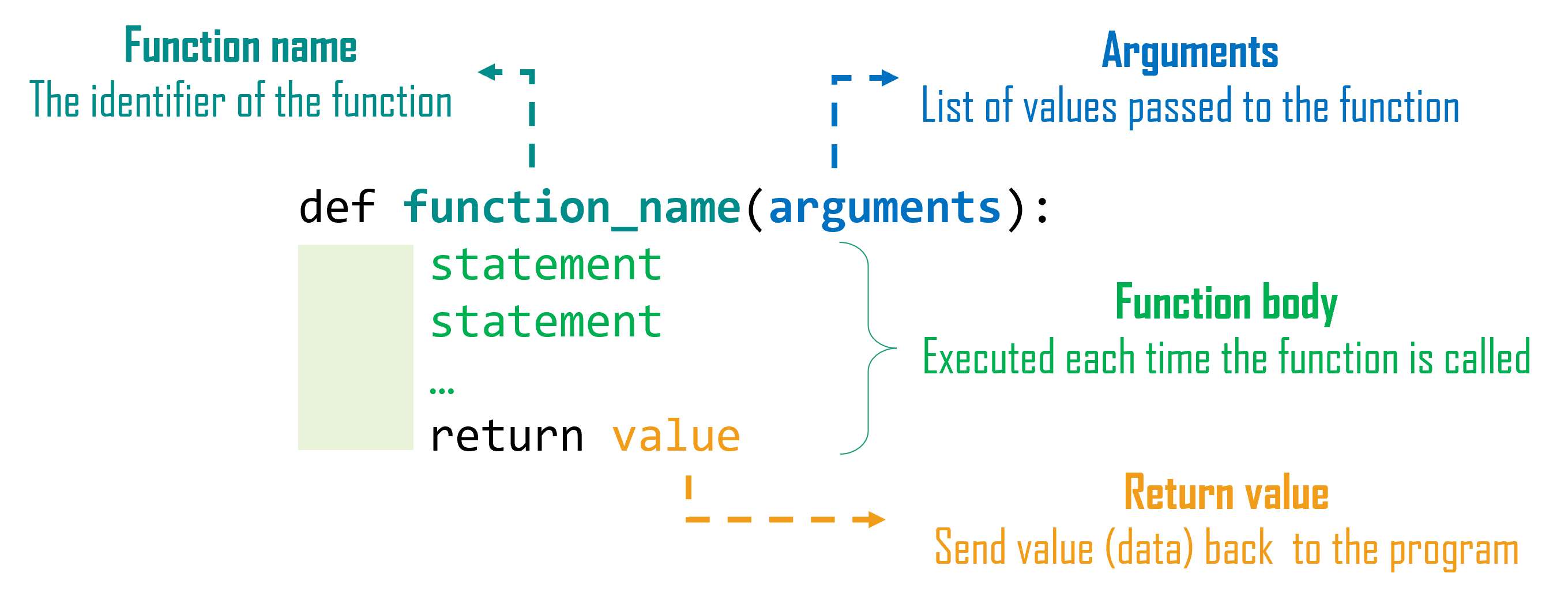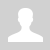Python. Functions (en)

11.3.1.1 write code in a programming language using functions
11.3.1.2 assign function parameters
11.3.1.3 define the scope of variables

# Python. Functions

A function in programming, or a subroutine, is a piece of program code that can be accessed from another place in the program.

A function is a specially grouped set of commands that are executed sequentially but considered as a whole. In this case, the function may return (or not return) its result.

Functions...

• allow not to write repetitive program code;
• improve the readability of the program;
• allow you to quickly fix errors in the program.

A function is declared before it is called. A function description begins with a command def, followed by the name of the function, and a list of arguments in parentheses. The arguments get their values from the main program. A colon is placed at the end of the function declaration line.Example,

```def name_of_function(arguments):     statement1     statement2     ...     return value # the function may return (or not return) its result # main program name_of_function(parameters) # function call```

.

Example

A function that prints the string "Hello!" and returns nothing

```def hello(): # creates a function with the identifier hello     print("Hello!") # the function output Hello! hello() # function call```

Passing Parameters to Function Arguments

When we call a function we can pass actual values or variable values as parameters

Example

```def greet(name): # greet function will take one argument     print("Hello,", name) # the function output Hello, Roman greet("Roman") # call function greet and pass value "Roman" to the argument name```

We can send several parameters. The value of the first parameter goes to the first argument, the value of the second parameter goes to the second argument, etc.

Example

```def mult(a, b, c): # mult function takes three arguments     return a * b * c  # returns the multiplication of three numbers print(mult(2, 3, 4)) # 2 --> a, 3 --> b, 4 --> c # output 24```

Setting default values

We can set default values for arguments. In this case, if we do not pass the parameter to the argument, then the default value of the argument is used, if we pass, then the value of the parameter is used.

Example 1

```def mult(a, b, c=1): # c has default value     return a * b * c print(mult(2, 3)) # 2 --> a, 3 --> b # output 6```

Example 2

```def mult(a, b=1, c=1): # b and c has default value     return a * b * c print(mult(2)) # 2 --> a # output 2```

Example 3

```def mult(a=0, b=1, c=1): # a, b and c has default values     return a * b * c print(mult()) # without parameters # output 0```

If we pass parameters to default arguments, then the arguments get the value from the parameters.

Example 4

```def mult(a=0, b=1, c=1): # a, b and c has default value, but a and b change values     return a * b * c # 3 * 5 * 1 print(mult(3, 5)) # 3 --> a, 5 --> b # output 15```

Arbitrary Arguments (*args)

If we do not know how many values will be passed to the arguments, then an arbitrary argument with an asterisk is used. Arbitrary argument packs values into a tuple.

Example

```def numbers(*nums): # *args     return nums  print(numbers(3, 5, 9)) # output (3, 5, 9) print(numbers(1, 7, 5, 4)) # output (1, 7, 5, 4)```

Keyword arguments (**kwargs)

A set of named arguments can be passed a keyword argument with two asterisks. Keyword argument packs values into a dictionary.

Example

```def capitals(**cities): # **kwargs     return cities print(capitals(Turkey="Ankara", Russia="Moscow")) # output {'Turkey': 'Ankara', 'Russia': 'Moscow'} print(capitals(China="Beijing", Austria="Vienna", Greece="Athens")) # output {'China': 'Beijing', 'Austria': 'Vienna', 'Greece': 'Athens'}```

Example using *args and **kwargs

```def func_nums(*nums, **words): # *args, **kwargs     return nums, words print(func_nums(5, 7, 10, 8, one=1, two=2, five=5))   # output ((5, 7, 10, 8), {'one': 1, 'two': 2, 'five': 5})```

Questions:

Exercises: